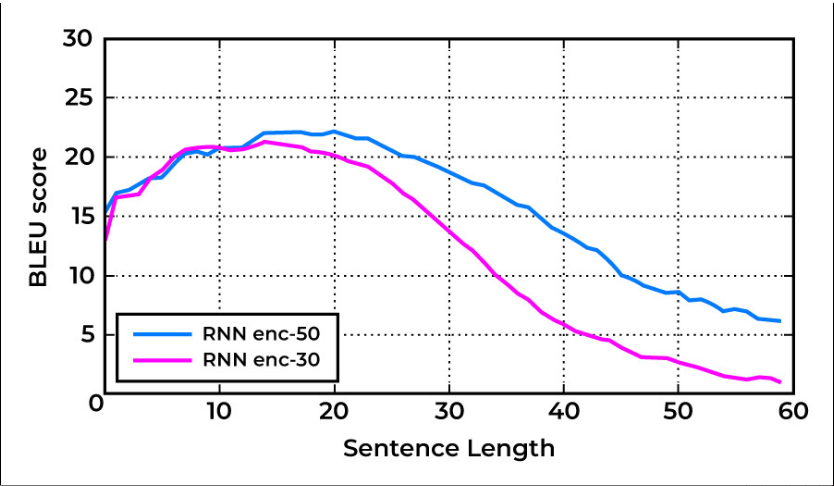Open in App
Not now

# NLP – BLEU Score for Evaluating Neural Machine Translation – Python

• Difficulty Level : Expert
• Last Updated : 26 Oct, 2022

Neural Machine Translation (NMT) is a standard task in NLP that involves translating a text from a source language to a  target language. BLEU (Bilingual Evaluation Understudy) is a score used to evaluate the translations performed by a machine translator. In this article, we’ll see the mathematics behind the BLEU score and its implementation in Python.

## BLEU Score

As stated above BLEU Score is an evaluation metric for Machine Translation tasks. It is calculated by comparing the n-grams of machine-translated sentences to the n-gram of human-translated sentences. Usually, it has been observed that the BLEU score decreases as the sentence length increases. This, however, might vary depending upon the model used for translation. The following is a graph depicting the variation of the BLEU Score with the sentence length.## Mathematical Expression for BLEU Score

In cases for BLEU Score translation,  Mathematically, BLEU Score is given as follows:Here,

BP stands for Brevity Penalty, which penalizes the score when the Machine Translation is too short compared to the Reference (Correct) translations.

n ∈ [1,4]

Pn is the n-gram modified precision score.

The mathematical expression for Brevity Penalty is given as follows:Pn can be defined as follows:For a better understanding of the calculation of the BLEU Score,  let us take an example. Following is a case for French to English Translation:

• Source Text (French): cette image est cliqué par moi
• Machine Translated Text: the picture by me
• Reference Text-1: this picture is clicked by me
• Reference Text-2: the picture was clicked by me

We can clearly see that the translation done by the machine is not accurate. Let’s calculate the BLEU score for the translation.

For n = 1, we’ll calculate the Unigram Modified Precision:

Here the unigrams (the, picture, by, me) are taken from the machine-translated text. Count refers to the frequency of n-grams in all the Machine Translated Text, and Clipped Count refers to the frequency of unigram in the reference texts collectively.For n = 2, we’ll calculate the Bigram Modified Precision:For n = 3, we’ll calculate the Trigram Modified Precision:For n =4, we’ll calculate the 4-gram Modified Precision:Now we have computed all the precision scores, let’s find the Brevity Penalty for the translation:• Machine Translation Output Length = 7 (Machine Translated Text: the picture the picture by me)
• Max Reference Output Length  = 7 (Reference Text-2: the picture was clicked by me)Finally, the BLEU score for the above translation is given by:On substituting the values, we get,Finally, we have calculated the BLEU score for the given translation.

## BLEU Score Implementation in Python

Having calculated the BLEU Score manually, one is by now accustomed to the mathematical working of the BLEU score. However, Python’s NLTK provides an in-built module for BLEU score calculation. Let’s calculate the BLEU score for the same translation example as above but this time using NLTK.

Installation Requirements:

pip install datasets transformers[sentencepiece]

Code:

## Python3

 from datasets import load_metric  bleu = load_metric("bleu")predictions = [["the", "picture", "the", "picture",                "by", "me"]]references = [    [["the", "picture", "is", "clicked", "by", "me"],      ["this", "picture", "was", "clicked", "by", "me"]]]print(bleu.compute(predictions=predictions, references=references))

Output:

{'bleu': 0.0,
'precisions': [0.6666666666666666, 0.4, 0.0, 0.0],
'brevity_penalty': 1.0,
'length_ratio': 1.0,
'translation_length': 6,
'reference_length': 6}

We can see that the BLEU score computed using Python is the same as the one computed manually. Thus, we have successfully calculated the BLEU score and understood the mathematics behind it.

My Personal Notes arrow_drop_up